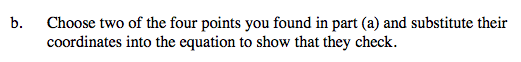### Home > A2C > Chapter 13 > Lesson 13.1.1 > Problem13-13

13-13.
1. Graph =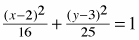. Homework Help ✎

1. What are the coordinates of the four relocated intercepts? These points, at the top, bottom, left, and right extremes of the ellipse, are called the vertices of the ellipse.

2. Choose two of the four points you found in part (a) and substitute their coordinates into the equation to show that they check.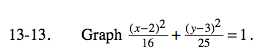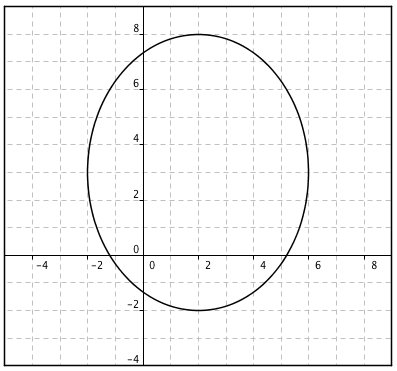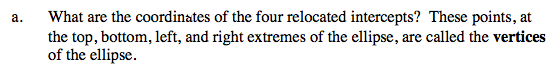Set one of the variables so that its squared quantity is zero, then solve for the other.

$\frac{((2)-2)^2}{16}+\frac{(y-3)^2}{25}=1$

(0) + (y − 3)3 = 25
y = 3 ± 5